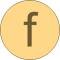# normalize

Return normalized vector such that length = 1 and prevent zero-division for zero vector# Information

This information is part of the Modelica Standard Library maintained by the Modelica Association.

#### Syntax

```Vectors.normalize(v);
Vectors.normalize(v,eps=100*Modelica.Constants.eps);
```

#### Description

The function call "`Vectors.normalize(v)`" returns the unit vector "`v/length(v)`" of vector v. If length(v) is close to zero (more precisely, if length(v) < eps), v/eps is returned in order to avoid a division by zero. For many applications this is useful, because often the unit vector e = v/length(v) is used to compute a vector x*e, where the scalar x is in the order of length(v), i.e., x*e is small, when length(v) is small and then it is fine to replace e by v to avoid a division by zero.

Since the function has the "Inline" annotation, it is usually inlined and symbolic processing is applied.

#### Example

```  normalize({1,2,3});  // = {0.267, 0.534, 0.802}
normalize({0,0,0});  // = {0,0,0}
```